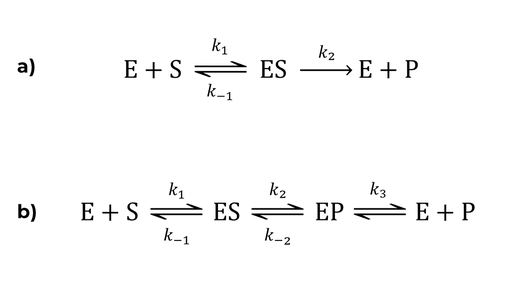The Michaelis-Menten equations, as you have seen in the previous section, describes the rate of a 1-substrate enzyme-catalyzed reaction. The parameters Vmax and Km can be obtained experimentally for any given enzyme; however, they themselves provide very little information about the reaction mechanism, such as the number of discrete steps and their individual reaction rates. The reaction rate (V) is defined as the change in concentration over time. The rate can be expressed as either the rate of formation of products (P) or as the rate of consumption of reactants (the substrate, S) .

V = d[P]/dt = -d[S]/dt

Note that the rate of consumption of the substrate is equal to the negative change in the concentration of the substrate over time. The Michaelis-Menten equation described on the previous page is based on the reaction mechanism in Figure 1.a:Figure 1: Figure 1.a; Overall enzymatic reaction. Figure 1.b; Extended enzymatic reaction.

This mechanism includes 3 individual reactions with three different rate constants:

• E + S → ES, formation of the enzyme-substrate complex, with the rate constant k1

• ES → E + S, dissociation of the enzyme and the substrate, with the rate constant k-1

• ES → E + P, dissociation of the enzyme and the product, with the rate constant k2

In the Michaelis-Menten model it is assumed that the third reaction is the rate-limiting step, and the associated rate constant k2 is also called the turnover number or kcat. For another reaction mechanism, the turnover number would be defined differently, for instance, in the reaction in Figure 1.b where the last step is the rate-limiting step. Here, kcat is equal to the rate constant of this step, i.e., kcat = k3. For reactions with more complicated reaction mechanisms, kcat can be a function of several rate constants. 

When measuring the reaction rate of a given enzymatic reaction, it is important to measure the initial reaction rate, which is the reaction rate at the beginning of the reaction.

1. Atkins, Peter W.; de Paula, Julio; Friedman, Ronald (2009). Quanta, Matter, and Change: A molecular approach to physical chemistry. Oxford University Press. ISBN 978-0-19-920606-3.

2. Lehninger, Albert L.; Nelson, David L.; Cox, Michael M. (2008). Principles of Biochemistry (5th ed.). New York, NY: W.H. Freeman and Company. ISBN 978-0-7167-7108-1.

Initial reaction rate

Theory overview